Related Articles

# Arithmetic Micro-operations in Registers

• Difficulty Level : Easy
• Last Updated : 19 Jul, 2021

We can perform arithmetic operations on the numeric data which is stored inside the registers.

Example :

`R3 <- R1 + R2`

The value in register R1 is added to the value in the register R2 and then the sum is transferred into register R3. Similarly, other arithmetic micro-operations are performed on the registers.

In addition micro-operation, the value in register R1 is added to the value in the register R2 and then the sum is transferred into register R3.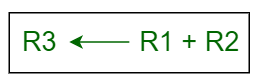• Subtraction –
In subtraction micro-operation, the contents of register R2 are subtracted from contents of the register R1, and then the result is transferred into R3.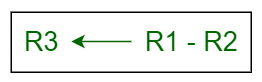There is another way of doing the subtraction. In this, 2’s complement of R2 is added to R1, which is equivalent to R1 – R2, and then the result is transferred into register R3.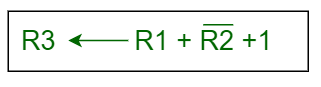• Increment –
In Increment micro-operation, the value inside the R1 register is increased by 1.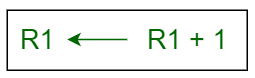• Decrement –
In Decrement micro-operation, the value inside the R1 register is decreased by 1.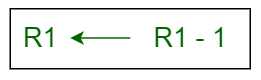• 1’s Complement –
In this micro-operation, the complement of the value inside the register R1 is taken.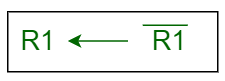• 2’s Complement –
In this micro-operation, the complement of the value inside the register R2 is taken and then 1 is added to the value and then the final result is transferred into the register R2. This process is also called Negation. It is equivalent to -R2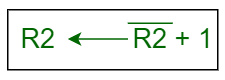Attention reader! Don’t stop learning now.  Practice GATE exam well before the actual exam with the subject-wise and overall quizzes available in GATE Test Series Course.

Learn all GATE CS concepts with Free Live Classes on our youtube channel.

My Personal Notes arrow_drop_up